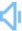Directly to word page Vague search(google)

## Divide in a sentence

Sentence count:265+20 Only show simple sentencesPosted:2016-07-16Updated:2020-07-24
Synonym: sortAntonym: Similar words: Meaning: [dɪ'vaɪd]n. 1. a serious disagreement between two groups of people (typically producing tension or hostility) 2. a ridge of land that separates two adjacent river systems. v. 1. separate into parts or portions 2. perform a division 3. act as a barrier between; stand between 4. come apart 5. make a division or separation 6. force, take, or pull apart.Random good picture Not show
1. Class differences can divide a nation.
2. Divide the cake with your brother.
3. I hope this disagreement does not divide us.
4. To divide a computer program into segments.
5. The cells began to divide rapidly.
6. Divide eight by two you get four.
7. Scientists traditionally divide the oceans into zones.
8. We'll divide the profit fifty-fifty.
9. His son is learning to multiply and divide.
10. Divide ten by two,and you get five.
11. All things invariably divide into two.
12. The children are already learning to multiply and divide.
13. To divide by 100 is done by only pointing off two figures for decimals.
14. The idea is to divide up the country into four sectors.
15. Let's divide the cost.
16. Think of me sometimes while Alps and ocean divide us,but they ever will,unless you wish it.
17. The leader's speech aimed to close the embarrassing divide in party ranks.
18. Latest statistics suggest the North/South divide is becoming even more pronounced.
19. For the sake of simplicity, let's divide the discussion into two parts.
20. Divide it in half.
21. Those cells divide and give many other different types of cells.
22. Scientists divide animals, plants and rocks into classes.sentencedict .com
23. The soldiers began to divide the spoils.
24. Divide up into two teams.
25. Equally divide profits between the partners.
26. Divide the cake into equal parts.
27. How shall we divide up the work?
28. The mountain is the divide between the two rivers.
29. Add the two numbers together and divide by three.
30. At the end of the lecture, I'd like all the students to divide into small discussion groups.
Total 265, 30 Per page  1/9  «first  next  last»  goto